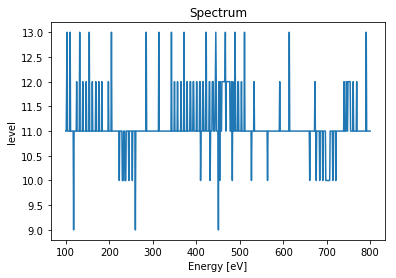This document gives detailed examples about spectrum calculations in OSCARS.

Any of these can be run in multi-threaded, GPU, or MPI mode. Results from running on separate nodes on grid/cloud computing can be combined.

## Polarization¶

One can select which polarization to compute. The default is 'all' polarizations. One may also specify the polarization parameter as:

• 'linear-horizontal' or 'lh'
• 'linear-vertical' or 'lv'
• 'circular-right' or 'cr'
• 'circular-left' or 'cl'

One may alternatively specify the angle of polarization of interest with respect to the horizontal direction as (for instance for 45 degrees):

• angle=45. * osr.pi() / 180.

Calculating polarization requires a definition of the horizontal and vertical directions. The defaults for these assume the beam is in the +z direction with +x being the horizontal direction. It is possible to change these definitions with the parameters horizontal_direction and propogation_direction

## What do you need to calculate a spectrum?¶

Specifically, all you need is a trajectory (position and velocity), an observer position, and a set of points (energy) where you are interested in the flux. Practically in OSCARS this usually means defining a beam, a magnetic field, calculating the trajectory, and calculating the flux for the points of interest.

When calling calculate_spectrum() npoints is an optional parameter and will default to a pitch of 1 eV or 100 points (whichever is greater).

In :
# matplotlib plots inline
%matplotlib inline

# Import the OSCARS SR module
import oscars.sr

# Import OSCARS plots (matplotlib)
from oscars.plots_mpl import *

OSCARS v2.1.8 - Open Source Code for Advanced Radiation Simulation
Brookhaven National Laboratory, Upton NY, USA
http://oscars.bnl.gov
oscars@bnl.gov

In :
# Create a new OSCARS object.  Default to 8 threads and always use the GPU if available

In :
# For these examples we will make use of a simple undulator field
osr.add_bfield_undulator(bfield=[0, 1, 0], period=[0, 0, 0.042], nperiods=31)

# Plot the field
plot_bfield(osr)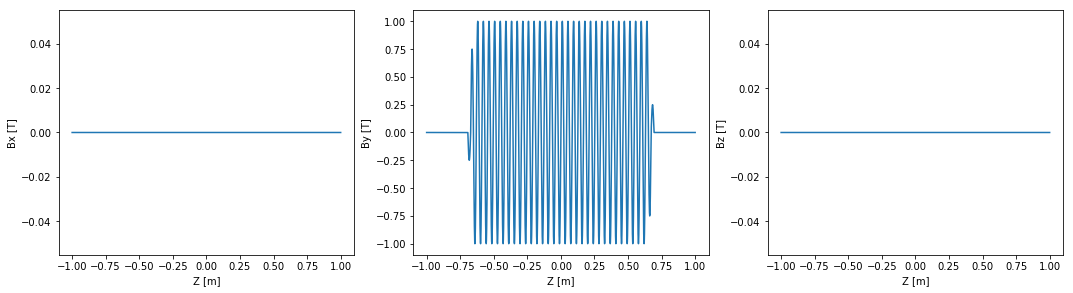## Single particle spectra¶

### Beam¶

Add a basic beam somewhat like NSLS2. Filament beam for simple studies.

In :
# Add a basic electron beam with zero emittance
osr.set_particle_beam(
energy_GeV=3,
x0=[0, 0, -1],
current=0.500
)

# You MUST set the start and stop time for the calculation
osr.set_ctstartstop(0, 2)

# Plot trajectory
plot_trajectory_position(osr.calculate_trajectory())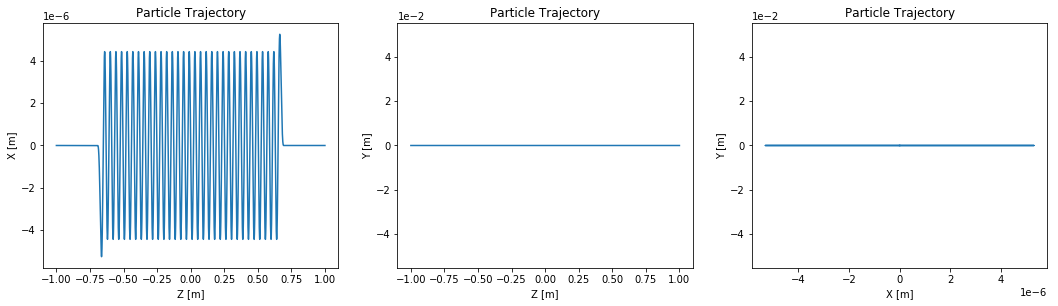### Spectrum - Energy Range¶

Calculate the spectrum in a given energy range with npoints evenly spaced.

In :
# Evenly spaced spectrum in an energy range
spectrum = osr.calculate_spectrum(obs=[0, 0, 30], energy_range_eV=[100, 800])
plot_spectrum(spectrum)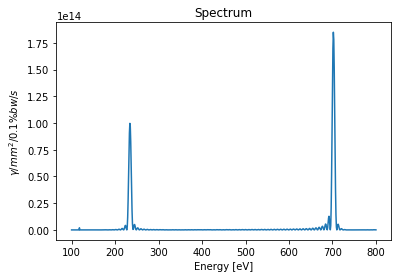In :
# Create a list of energy points you are interested (Skipping a region we are not interested in)
my_energy_list = []
my_energy_list.extend(list(range(200, 300)))
my_energy_list.extend(list(range(650, 750)))

# Evenly spaced spectrum in an energy range
spectrum = osr.calculate_spectrum(obs=[0, 0, 30], points_eV=my_energy_list)

# Here add plt.plot argument to show points (any extra arguments are passed to matplotlib)
plot_spectrum(spectrum, marker='.')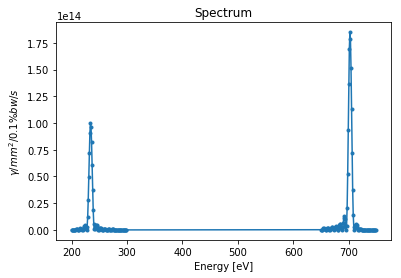In :
# You can also do the above in the following way by calculating 2 spectra and plotting
spectrum0 = osr.calculate_spectrum(obs=[0, 0, 30], points_eV=list(range(200, 300)))
spectrum1 = osr.calculate_spectrum(obs=[0, 0, 30], points_eV=list(range(650, 750)))

plot_spectra([spectrum0, spectrum1], ['200-300 [eV]', '650-750 [eV]'])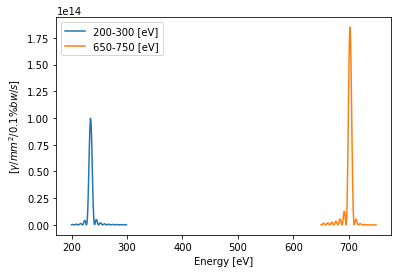### Spectrum - Polarization¶

Calculate the spectrum for different polarization modes

In :
# Horizontal, circular-right, and 30 degrees from the horizontal
spectrum_lh  = osr.calculate_spectrum(obs=[0, 0, 30], energy_range_eV=[200, 300], polarization='lh')
spectrum_cr  = osr.calculate_spectrum(obs=[0, 0, 30], energy_range_eV=[200, 300], polarization='cr')
spectrum_deg = osr.calculate_spectrum(obs=[0, 0, 30], energy_range_eV=[200, 300], angle=30 * osr.pi()/180)

plot_spectra([spectrum_lh, spectrum_cr, spectrum_deg],
['linear-horizontal', 'circular-right', '30-degrees from LH']
)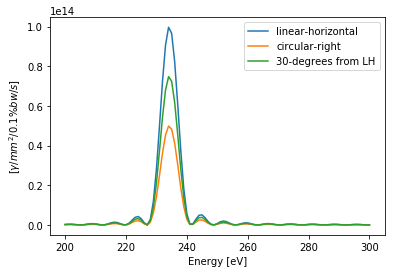## Multi-particle spectra¶

### Non-zero emittance beam¶

In :
# Add a basic electron beam with zero emittance
osr.set_particle_beam(
energy_GeV=3,
x0=[0, 0, -1],
current=0.500,
sigma_energy_GeV=0.001*3,
beta=[1.5, 0.8],
emittance=[0.9e-9, 0.008e-9]
)

# You MUST set the start and stop time for the calculation
osr.set_ctstartstop(0, 2)


### Ideal particle spectrum from non-zero emittance beam¶

If you don't set the ideal particle you will get a randomized particle...

In :
# Evenly spaced spectrum in an energy range
osr.set_new_particle(particle='ideal')
spectrum_ideal = osr.calculate_spectrum(obs=[0, 0, 30], energy_range_eV=[100, 800], npoints=701)
plot_spectrum(spectrum_ideal)### Multi-particle spectrum from non-zero emittance beam¶

To enable multi-particle mode add to the calculation: nparticles=1234 where 1234 is the number of particles you want to use in the simulation.

In :
# Evenly spaced spectrum in an energy range
spectrum_multi = osr.calculate_spectrum(obs=[0, 0, 30], energy_range_eV=[100, 800], npoints=701, nparticles=50)
plot_spectrum(spectrum_multi)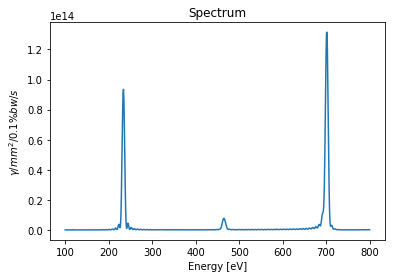### Plot spectra together¶

use the 'plot_spectra' function from oscars.plots_mpl

In :
plot_spectra([spectrum_ideal, spectrum_multi], ['ideal', 'multi'])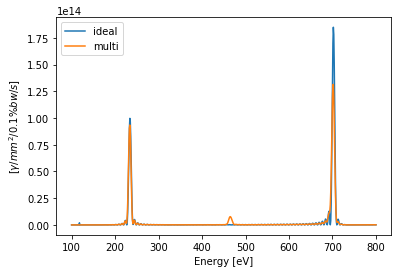## Spectrum as a function of position¶

Just for fun, let's say you were interested in the spectrum as a function of the observer position. Let's list of points scanning from the center outwards in the vertical direction.

In :
# Create list of observation points
obs_list = []
for y in range(0, 5):
obs_list.append([0, y * 2e-3, 30])

# Evenly spaced spectrum in an energy range
spectrum_list = []
label_list = []
for obs in obs_list:
osr.set_new_particle(particle='ideal')
spectrum_list.append(osr.calculate_spectrum(obs=obs, energy_range_eV=[100, 800]))
label_list.append('y=' + str(obs*1000) + ' [mm]')

plot_spectra(spectrum_list, label_list)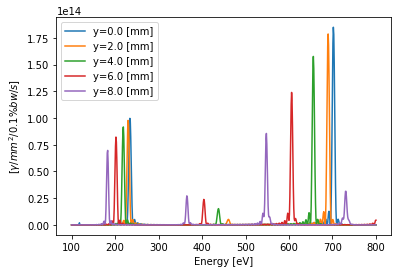## On Precision¶

The default relative precision is 0.01 (1%) and is controlled by the parameter:

• precision=0.01 (default)

You may retrieve the relative precision for all points in a calculation by including the parameter:

• quantity='precision'

Should you not reach the desired precision withing max_level you will receive a warning message. To increase max_level you have two options:

• max_level=25
• max_level_extended=(some number above max_level)

The maximum max_level is 25 due to typical memor restrictions (because it is faster). The 'extended' version runs in non-memory mode which allows higher precision at the cost of CPU time. Only in rare instances will you need this. You can also retrieve the 'level' of convergence for all points (which will show -1 for non-converged points) with the addition of:

• quantity='level'
In :
# Show the precision reached for each point
spectrum = osr.calculate_spectrum(
obs=[0, 0, 30],
energy_range_eV=[100, 800],
quantity='precision'
)
plot_spectrum(spectrum, title='Precision', ylabel='Precision')

# As a test, increase the precision from previous
spectrum = osr.calculate_spectrum(
obs=[0, 0, 30],
energy_range_eV=[100, 800],
precision=0.001,
quantity='precision'
)
plot_spectrum(spectrum, title='Precision', ylabel='Precision')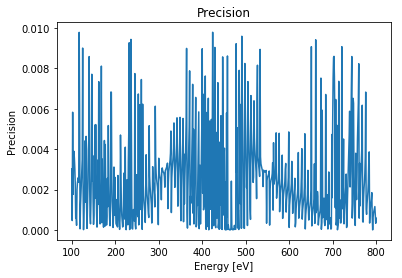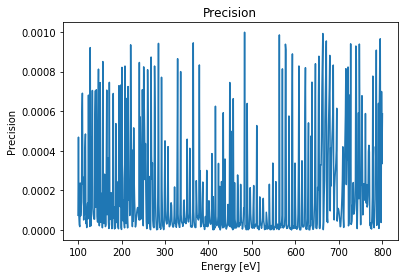In :
# Show the 'level' reached for each point
spectrum = osr.calculate_spectrum(obs=[0, 0, 30], energy_range_eV=[100, 800], quantity='level')
plot_spectrum(spectrum, ylabel='level')